# Theory of (organic) (thin film) Field-Effect Transistors

The idea is here to present the theory of organic field effect transistors. I wil try to do this in a very simplified way, cutting away all the details. However, I am well aware that in this way, I may oversimplify things. This should serve as an introduction to get a feeling for electronic devices. This guide does not substitute serious books like Sze and Shur, whose reference can be found at the end of this document.

To explain how a field-effect transistor works, we start with a thick-film MOS-FET (metal-oxide-semiconductor field-effect transistor) or a more general MIS-FET when the insultaor is not silicon-oxide. As we will see, the MIS-FET can be well described by a MIS-diode with source and drain electrodes connected in the plain of the active layer. Therefore, it is best to start with the MIS juntion. Then we can extend this theory to finally arrive at the accumulation-channel FET:
::: MIS junction.
::: Capacitance of an MIS junction
::: Field-Effect transistor (inversion channel)
-- Linear region
-- Threshold voltage of an inversion-channel FET
-- Subthreshold region of an inversion-channel FET
-- Saturation
-- Contact resistance
-- Other contact effects
-- Stress
::: Organic FETs
-- Accumulation-channel FET

Peter Stallinga, June 2004.

## MIS junction

To understand how an FET (of the MOSFET type) works, we first have to analyze what an MIS structure is.
In a simple approximation, a MOSFET is a planar device of semiconducting material whose free carrier concentration n, and hence it conductivity (via g = men) is controlled by the gate.

The first figure shows an energy diagram of an MIS device. The device consists of a metal and a semiconductor separated by an insulator. Here we assume that the insulator is not conducting any current.
Standard textbooks start with an ideal MIS device, which means that the Fermi levels in the metal and the semiconductor are aligned even before contact. In other words, the work functions of the metal and of the semiconductor are equal, fm = c + EC-EF. This implies that we have no band bending in the absence of external voltages. Remember the Schottky barrier, where charge was flowing from one side to the other due to the misalignement of the Fermi levels. In the ideal MIS junction such a flow of charge is not needed (which saves us the trouble of explaining how it can pass through the oxide :-)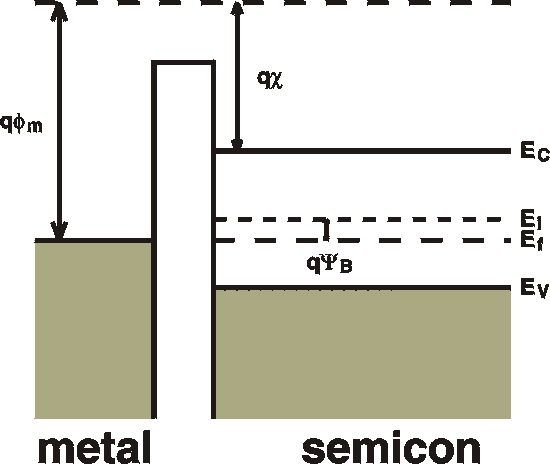fm : the metal workfunction. How much it costs to take an electron from the top of the sea of electrons in the metal to vacuum. The "workfunction" of the semiconductor can be defined as c + EC-EF. fb : (not shown, beause it doesn't enter into the calculations): the barrier height of the oxide as seen from the metal; the difference between the "conduction band" of the oxide and the Fermi level of the metal.. c : the electron affinity of the semiconductor. The energy it takes to take an electron from the conduction band to the vacuum level. EG : energy gap of the semiconductor. YB : the energy from the midgap level to the Fermi level. This is approximately equal to EG /2 EC : bottom of conduction band. EV : top of valence band.

 The following strip explains what happens when we connect an external voltage to the metal (gate). For that we use the legenda as shown here on the right. Note that the (horizontal) SCALE of the pictures is changing. Just like in Schottky barriers, the depletion width depends on the voltage. Here the depletion width is shown as constant.a) DepletionWhen a small (positive) voltage is connected to the metal (gate), free holes are pushed out of the interface region and a band bending results on the other side of the insulator. This is the same effect as seen in a Schottky barrier. Free carriers (holes) flow out of the interface region and a depletion zone is formed. The uncompensated (negatively) ionized acceptors cause an electric field and - via Poissons equation - a parabolic bending of the bands. Note that there is also a voltage drop in the oxide. In the oxide no charges can reside and hence the field is constant and the voltage drop is linear in space. The total voltage drop in the oxide plus the band bending is equal to the external voltage. b) Inversion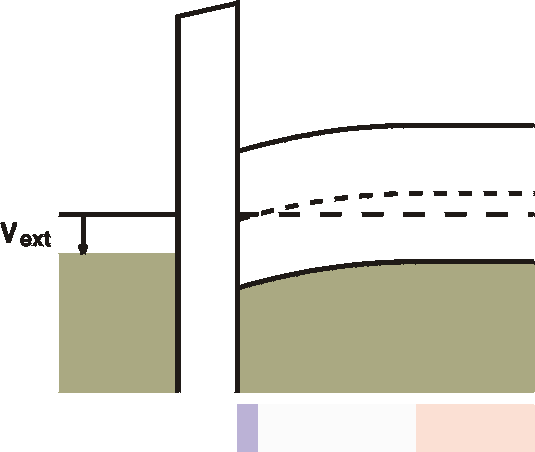When the voltage is further increased, at the interface an inversion region is created. The semiconductor becomes here n-type, albeit not very conductive, yet. c) Strong Inversion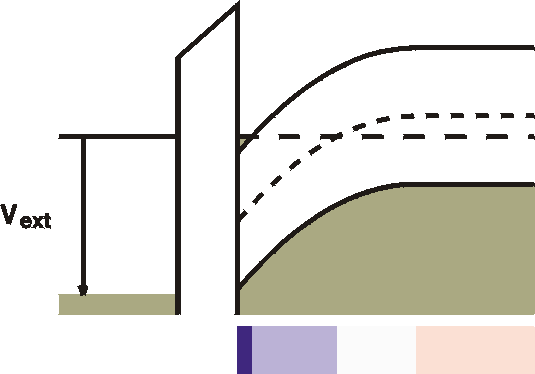For very strong bias, the Fermi level crosses the conduction band close to the interface. This is strong inversion. Free electrons are in a so-called "channel" next to the oxide. This channel is therefore highly conductive. The ample availability of states in the conduction band means that further increases in the gate voltage will not extend this strong inversion region into space, but rather will increase the density of electrons in the channels. The channel is always infinitisimally thin.The huge amounts of free carriers can easily cause a large voltage drop (band bending) and only a thin layer is needed to "absorb" the external voltage. Note that the depletion width (the white zone) and the inversion width (lightblue zone) are constant once the channel is formed. d) Accumulation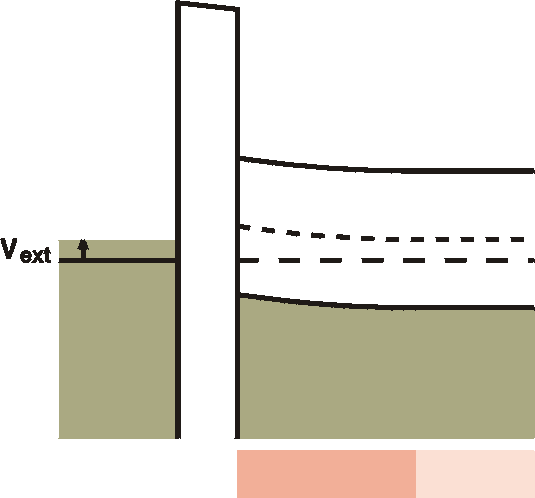Going in the other direction, a negative voltage at the gate metal will attract some free holes to the interface. In this accumulation region, an increased number of free holes is created, although it is not yet dramatic; the interface is still high-ohmic. e) Strong accumulationFor stronger negative voltages at the gate the Fermi level is forced below the valence band at the interface. A channel with high density free holes is created. This is called strong acummulation. Again, as for the strong inversion case, further increasing the voltage will result in an increase of the density of the free holes rather than an increase of the channel in space. In the picture the width of the channel is exaggerated. In reality it is only a monolayer thick.

To complete the story, in strong inversion we have the following distribution of space charge and free carriers: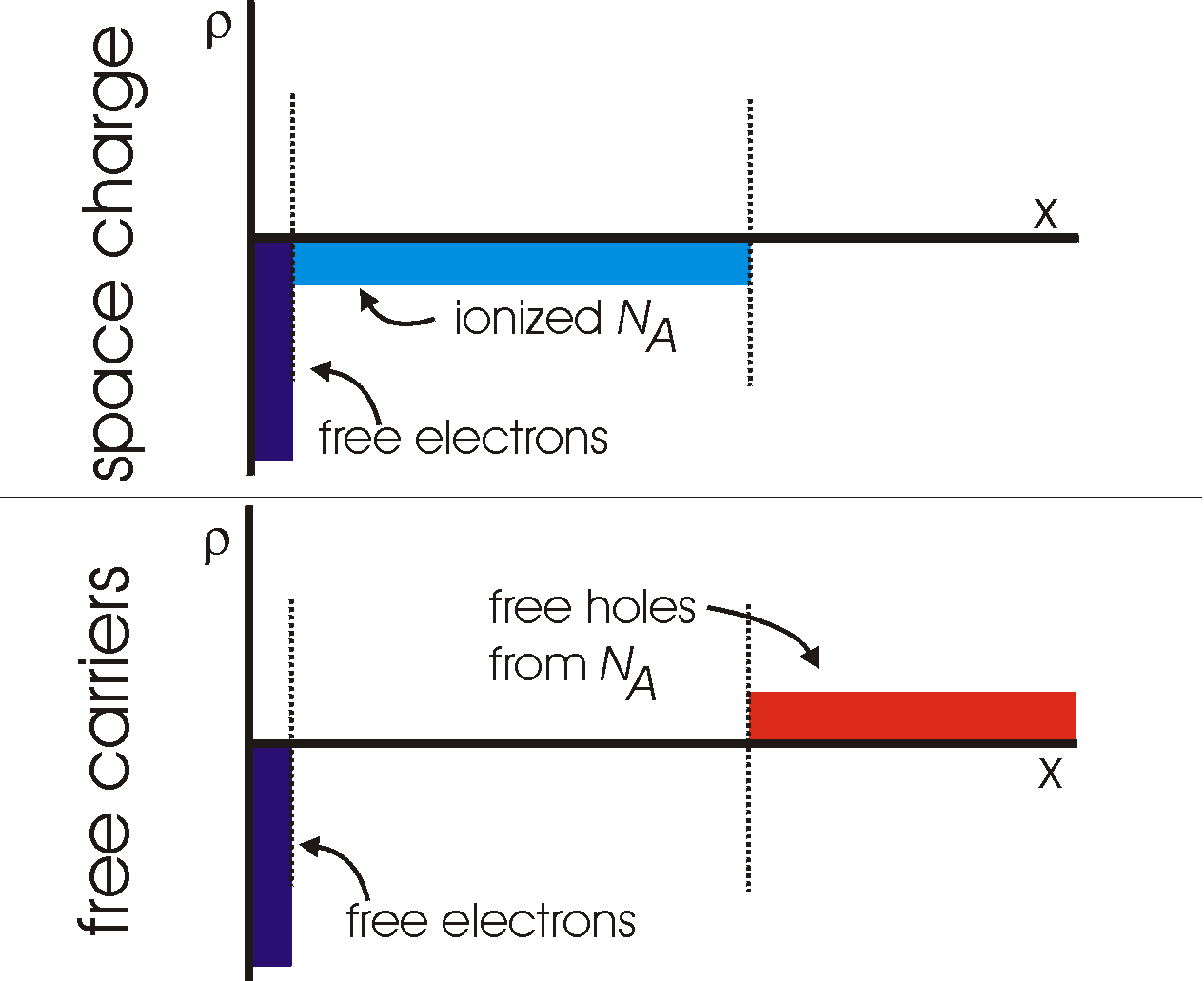The MIS junction is an ideal device for studying interface states and deep levels using the same techniques as demonstrated for the Schottky barrier. The advantage lies in the fact that there is no DC current which might obscure our measurements.

## Capacitance of an MIS junction:

The device can consist of several regions in series, each with it's own capcitance. The capacitance of the metal and of any part of the semiconductor with free carriers is zero. For the other two parts of the device, the insulator and the depletion region we can calculate:
Cox = eox / d
CW = es / W
The total capacitance is caluclated by taking the serial sum of the capacitances:
 Cox x CW depletion:       Cd = ----------- accumulation:  Ca = Cox Cox + CW
In acuumulation there is no depletion layer and in this case, the total capacitance reduces to Cox. Once a depletion zone is formed, the capacitance depends on the frequency. For low frequencies, the generation-recombination current causes a short circuit of the depletion capacitance and the capacitance reduces to Cox. See Fig. 7 on p. 371 of Sze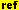or Fig. 4-3-5a on p. 348 of Shur. For high frequencies, the depletion zone is not short circuited and the measured capacitance is a series capacitance of the oxide and the depletion width. The depletion width is constant once it is fully formed (see images above) and we will measure a constant capacitance.

## Field Effect Transistor (FET)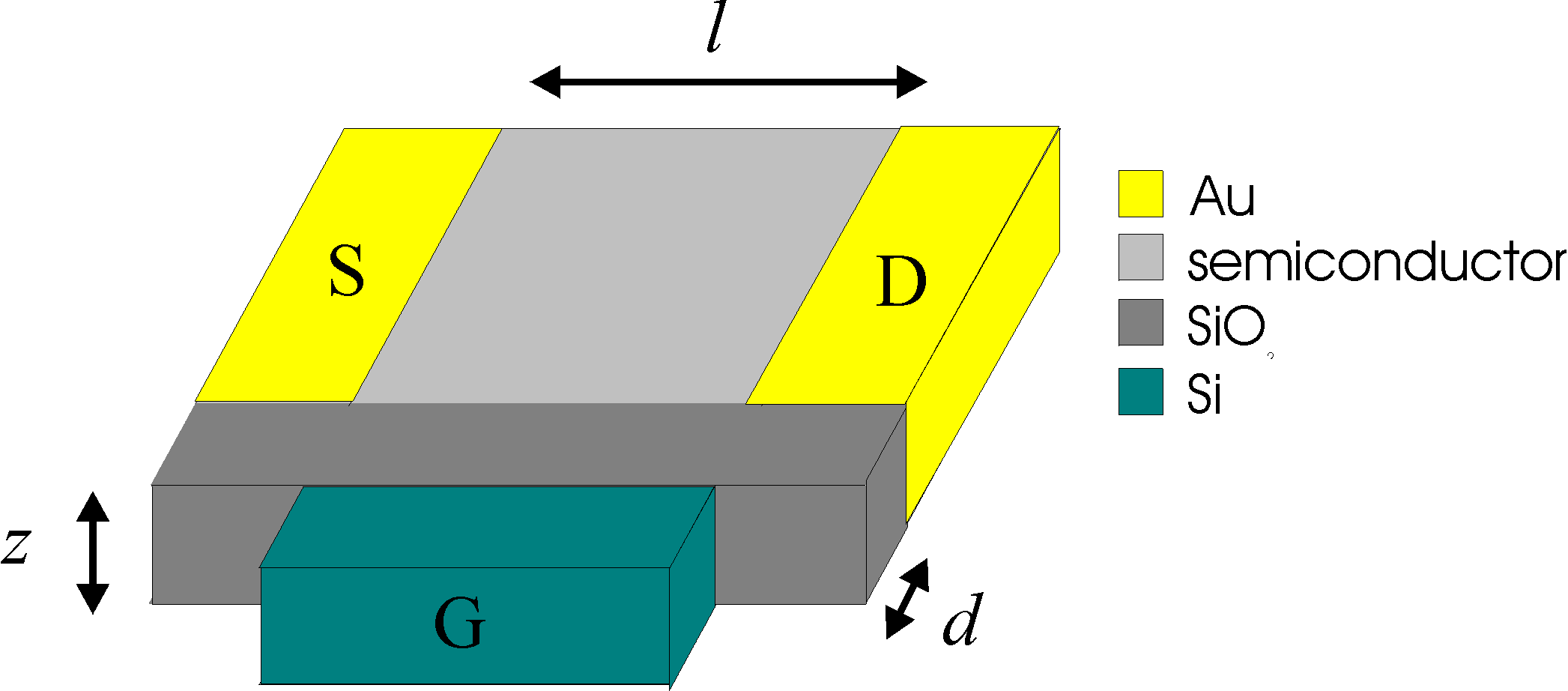Directions: x: from oxide to surface of film y: from source to drain z: along an electrode Z: electrode width L: electrode distance d: oxide thickness
An FET is nothing more than an MIS device with electrodes connected on two lateral sides of the semiconductor. Imagine connecting an electrode above and one below the images above. The first one we will call the source, the latter the drain.
The total current through this device is then linearly proportional to the number of free carriers in the semiconductor. The other parameters are the mobility m, the external electrical field Ex: and the device dimensions Z and L:
IDS    = Z|Q|mEx = Z|Q| mVy/L      ( I )
with IDS the total current, m the mobility of the carriers, Vy the external voltage (is equal to VDS), Z the width of an electrode, L the distance from source to drain, and |Q| the amount of free charge in a line perpendicular to the oxide surface (along x) in C/m2. This is equal to integrating the electron and hole density (ignoring the sign) from the edge of the oxide to infinity (or to the limits of the semiconductor film), see the figure with free carriers above.
In case we have substantial contributions from both electrons and holes, and they have different mobilities, we have to replace the part |Q|m with (|Qp|mp + |Qn|mn).
We will see that this can explain the linear region of an FET. To calculate the IV curves in the linear region, we only have to calculate the number of free carriers. For the saturation region the equations are a little different, as will be shown later.

### Linear region

As a first order we can say that we only have free carriers when we are in strong inversion or strong accumulation. As can be seen from the strip of figures above, we need to supply a certain voltage to the gate to induce either strong inversion or strong accumulation.This is the so-called threshold voltage VT. This doesn't mean that we don't have free carriers and conduction below this threshold voltage, but that the conduction beyond this voltage is much larger.

Under the assumption that all the free carriers come from charges in the strong accumulation or depletion zone, it is very easy to calculate the amount of charge on the semiconductor side and hence the current. In the section above we have seen that the capacitance of an MIS junction under these conditions is equal to the oxide capcitance, Cox. Assuming no other capacitances, all the charges go to the interface and therefore, since C = Q/V we find Q   =   Cox VG. As written above, we need VT to bring it into strong accumulation or depletion, therefore Q   =   Cox (VG -VT). With this in mind, and with the idea that the conduction is proportional to the charge as seen in equation I the current in the linear region becomes

 IDS    = Cox (VG -VT) mVDS Z/L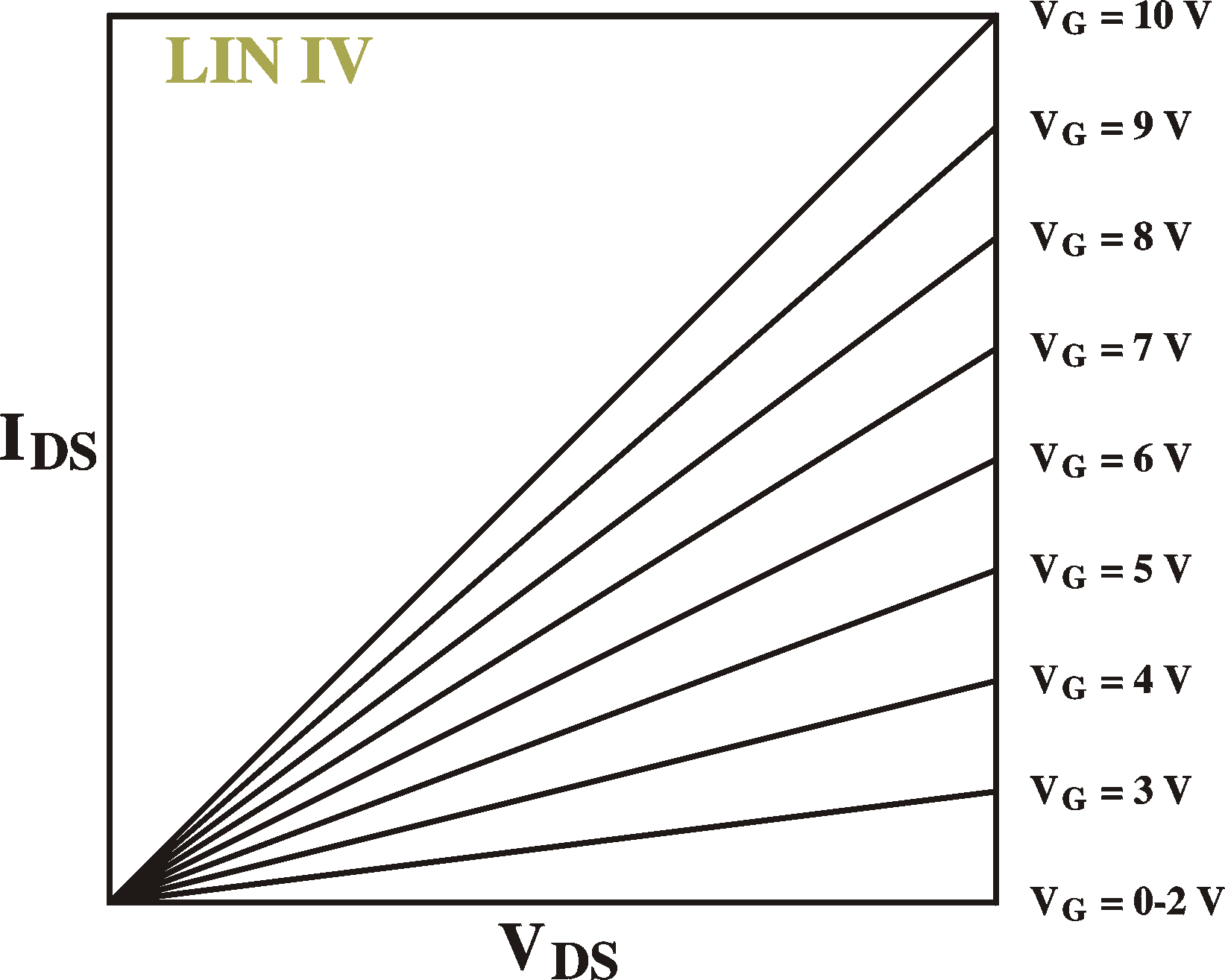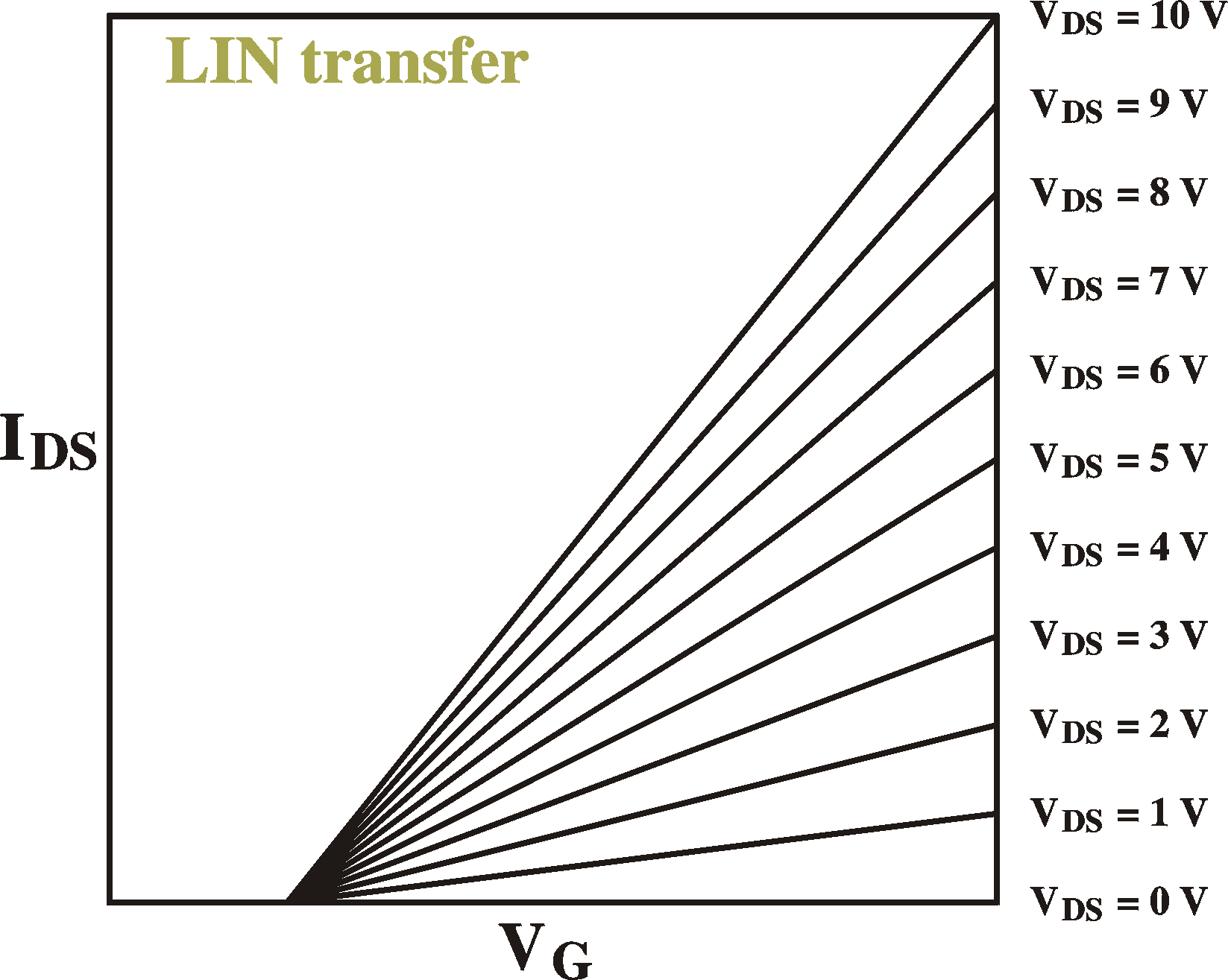Examples of IV curves (IDS vs. VDS; left) for different gate voltages and transfer curves (IDS vs. VG; right) for different drain-source voltages. VT = 2 V.

Remember that we arrived at this by the assumption that all the free carriers are located in the strong inversion or accumulation zone close to the interface and that the rest of the device is not conducting. Especially in materials with bulk Fermi levels close to the band edges, the background conductivity (the "off current") can be very large. In principle, the purer the material, the closer the Fermi level will be to mid gap and the less off-current we will have. Making the films thinner also helps.
Moreover, not all the charge induced by the gate is free charge. The above model is also known as the Charge Control Model. If we do include the background conduction in the bulk and the depletion and inversion zones, we have to add a term to the above equation, to arrive at the Schottky model, see Szeand Shur.

### Threshold voltage of an inversion-channel FET

Following the above discussion and the MIS strip of figures, it is clear that the band bending cannot be larger than the bandgap before either strong accumulation or strong inversion occurs. In other words, at the onset of strong inversion, the band bending Vbb is exactly equal to the difference between the conduction band and the Fermi level, (EC-EF)/q in the bulk.The depletion width is then (see section on Schottky barrier) W = (2esVbb/ qNA)1/2. The electrical field at the interface is the integral of the space charge inside this depletion region: Emax = (qNA/es)W = (qNA2Vbb / es)1/2.
Maxwell's equations tells us that, in the absence of any charge, the displacement D (=eE) is continous accross the interface. On the semiconductor side of the junction we have Ds = es(qNA2Vbb/ es)1/2. In the oxide we therefore have an electrical field of Eox = (1/eox)(qesNA2Vbb)1/2. This field is constant inside the oxide because there are no net charges there. We therefore have an extra voltage drop of DV = doxEox = (dox/eox)(qesNA2Vbb)1/2 accross the oxide. The total external voltage then becomes (dox/eox)(qesNA2Vbb)1/2 + Vbb = VT.. With Vbb equal to (EC -EF)/q this becomes
V  =  (dox/eox)[2qesNA(EC-EF)/q]1/2 + (EC-EF)/q
Remember that Cox = eox/dox, and, in normal cases, the Fermi level is very close to the valence band, so that (EC-EF)/q = EG/q which we can call 2yB. With this in mind, the above equation becomes the following text book equation for an n-type inversion channel:
 VT   =  (4qesyBNA)1/2 / Cox + 2yB

As an example: for NA = 1x1016 cm-3, Cox = 50 mF/m2, EG = 2.5 eV (yB = 1.25 V), es = 5.0e0 we get VT = 11.9 V + 2.5 V = 14.4 V; since the first term in the equation comes from the oxide and the second term comes from the semiconductor we can see that most of the external voltage (83%) is absorbed by the oxide (remarkably).
The Fermi level, of course, also depends on the acceptor concentration, so in total we get a complex dependence on NA. In textbooks, it is assumed that all acceptors are ionized. In fact, for organic materials, where the acceptor level can be very deep (but abundant) this is not necessarily the case. In fact, we should read NA as "ionized levels" and this can be a fraction of the real number of acceptors, unlike in classical materials such as Si and GaAs. Moreover, the fraction of levels ionized can be changed by changing the band bending (by applying a gate voltage) and thus, the threshold voltage can change when putting the device in operation. Depending on the depth of the levels, these changes can be in the order of seconds, to minutes and hours and even days.
Note that high levels of current have no effect on this trapping of charges, since they do not attribute to a different band bending or extra charges in the interface.
Note that for an accumulation type FET this calculation does not work because we do not have a space-charge region caused by ionized acceptor levels; VT does then not depend on NA., but only on the Fermi level and the number of valence band states.

Remark: We have considered here an FET without a wire connected to the bulk of the device. If we do so, the threshold voltage reduces to V  =  (2qes(yB-Vsub)NA)1/2 / Cox + 2yB, with Vsub the voltage of the substrate.

### Subthreshold region of an inversion-channel FET

The subtreshold region is the region of gate voltages before the channel is opened. The desnity of free carriers at the interface is exponentially depending on the distance between the conduction band and the Fermi level. When the channel is formed, the Fermi level remains fixed (resonant) at the edge of the conduction band. Further increases in voltage result only in a tiny shift of EF; only minute changes in EF are needed to create free charges Cox(VG-VT). In the subthreshold region the distance EF-EV is determined by the gate voltage and the acceptor concentration in the bulk in the following way (very similar to the calculation of the threshold voltage above):
1) Poisson's equation tells us that the band bending in the semiconductor is equal to double-integral of the density of ionized acceptors: Vbb = double-integral NA
2) This will tell us the field at the interface and hence
3) The field and voltage drop in the oxide and hence the
4) Total voltage drop in the device.
5) Reversing these calculations, if we know the total voltage accross the device, we will also know the band bending in the semiconductor.
6) If we know the band bending in the semiconductor and we know the initial distance between Fermi level and conduction band, we will know the position of the Fermi level at the interface.
7) If we know the position of the Fermi level at the interface, we know the density of free carriers at the interface (Fermi-Dirac distribution, which is more or less exponential)

If you do the calculation correct, you will see that the distance of the Fermi level depends linearly on the gate voltage and with a square-root on the acceptor density NA. Hence the current follows something like (Sze, Szur)

Ids = I0 exp[VGNA]

### Saturation

If we increase the drain-source voltage eventually we will go into saturation where the current becomes independent of the drain-source voltage It is very easy to understand why this should happen. Imagine an FET with a threshold voltage of VT = 1 V. We will apply a gate voltage well beyond this value, let's say 10 V. The channel is therefore open. For low drain-source voltages, both the drain and the source and hence the entire bulk region of the device is at 0 V, or close to 0 V at all places. At the drain, the gate-drain voltage is 10 V and this is well beyond the threshold voltage. Here we have a conducting channel. At the source (which is always at 0 V), the gate-source voltage is also 10 V and here the channel is also open. Actually, at all places do we have a conductive channel.
Let us now set the drain voltage to 10 V. At the source we still have a gate-source voltage of 10 V and the band bending here will still induce a channel. However, at the drain, the gate and the drain are at the same voltage; the gate-drain voltage is 0 V and this is below the threshold voltage for creating a conductive channel. The channel is closed here. This we call pinch-off.

If we assume a linear voltage drop from source to drain from 0 to 10 V, we can exactly calculate where the channel closes, namely at 10% distance from the drain. We have to bear in mind, though, that a closed channel has less free carriers and thus has higher resistivity. The law of continuity tells us that the current at every place of the device must be equal. Therefore, since voltage drop is current times resistivity, the voltage drops much faster in a closed-channel region than in a open-channel region. Moreover, if we assume that the free-carrier density in the closed-channel region is much smaller than the open-channel region, the pinch-off region is very small.

The voltage of start of saturation is easy to calculate. At this voltage the gate-drain voltage is exactly equal to the threshold voltage. VG-VD = VT. Thus (VS = 0, VD = VDS)

onset of saturation: VDS = VG-VT

Beyond this voltage, the current is constant. We will have a region from the source to near the drain with a voltage drop of VG-VTand a very thin region close to the drain with the rest of the voltage drop of VDS. The length of the first region is nearly independent of the total external voltage. It therefore has a contant length and voltage drop, and the current through it is therefore independent of VDS. Again, continuity tells us that the current at every point is equal, therefore, the saturation current is independnet of VDS.

With this we can calculate the free carrier density Q(x) and the voltage V(x) of every point in the channel at the onset of saturation. Along the way we will also calculate the current which is the current in saturation.
Imagine putting up a screen at a distance x from the source, peprpendicular to the source-drain direction, stretching as long as the electrode lengths (Z) and as high as the film thickness. We can calculate the current I through such a screen.
The current I at a crossection at a certain point x is equal to the free-charge density at that point Q(x), the carrier mobility m and the local field E(x) = dV(x)/dx:
I(x) = ZmQ(x) dV(x)/dx
Because of continuity, this current has to be constant along x and equal to the drain-source current IDS. The above equation then reduces to
dV(x) / dx = IDS/ZmQ(x)         (eq.I)
The free-carrier density is a function of the local field VG-V(x), as seen before:
Q(x) = Cox[VG-V(x)-VT]       (eq.II)
The solution to this system of diferential equations is easy (take the derivative of the second equation and put this in the first), to which we add the following boundary condition:
1a: the charge at the drain is zero: Q(L) = 0.
1b: The voltage at the drain is just enough to close the channel: V(L) = VG-VT.The soulution is then     Q(x) = [(2CoxIDS/Zm)(L-x)]1/2     V(x) = (VG-VT) - [(2IDS/mZCox)(L-x)]1/2 Using the next boundary condition    2a: The voltage at the source is 0: V(0) = 0    2b: The charge at the source is Q(0) = Cox (VG-VT) we can calculate the current

 IDS = (1/2)m(Z/L) Cox (VG-VT)2

which is the current at the onset of saturation and, since, as we have shown before, the current beyond this point is independent of VDS, this is the current in saturation (as long as VG < VDS + VT).
The total amount of charge stored in the device in saturation can be found by integrating the equation for the charge distribution above and multiplying by the device width Z:
at saturation, VDS = VG-VT:      Q = (2/3) Z (VG-VT) Cox
in the same way:
at VDS = 0 V :           Q = Z (VG-VT) Cox
Note: when we increase the bias, charge is coming out of the device. We have to be careful in our IV measurements that we do not measure the current of these charges going into and coning out of the device. See the section on displacement current.

We can also use the same method to calculate the current before saturation. Going back to the general solution of the system of differential equations:
V(x) = (VG-VT) - [(2IDS/mZCox)(x0-x)]1/2
As boundary conditions we now take:
1: The voltage at the source is 0; the charge is Cox (VG-VT)
2: The voltage at the drain is V(L) = VDS
The result is

 IDS = m(Z/L) Cox [(VG-VT)VDS - (1/2)VDS2]
which is equal to what we found for the linear region, except for the term VDS2 which becomes important only when we approach saturation. When we susbtitute VDS << VG-VT we get the equation for the linear region. When we substitute the saturation condition VDS = VG-VT we reproduce exactly the equation for saturation currents.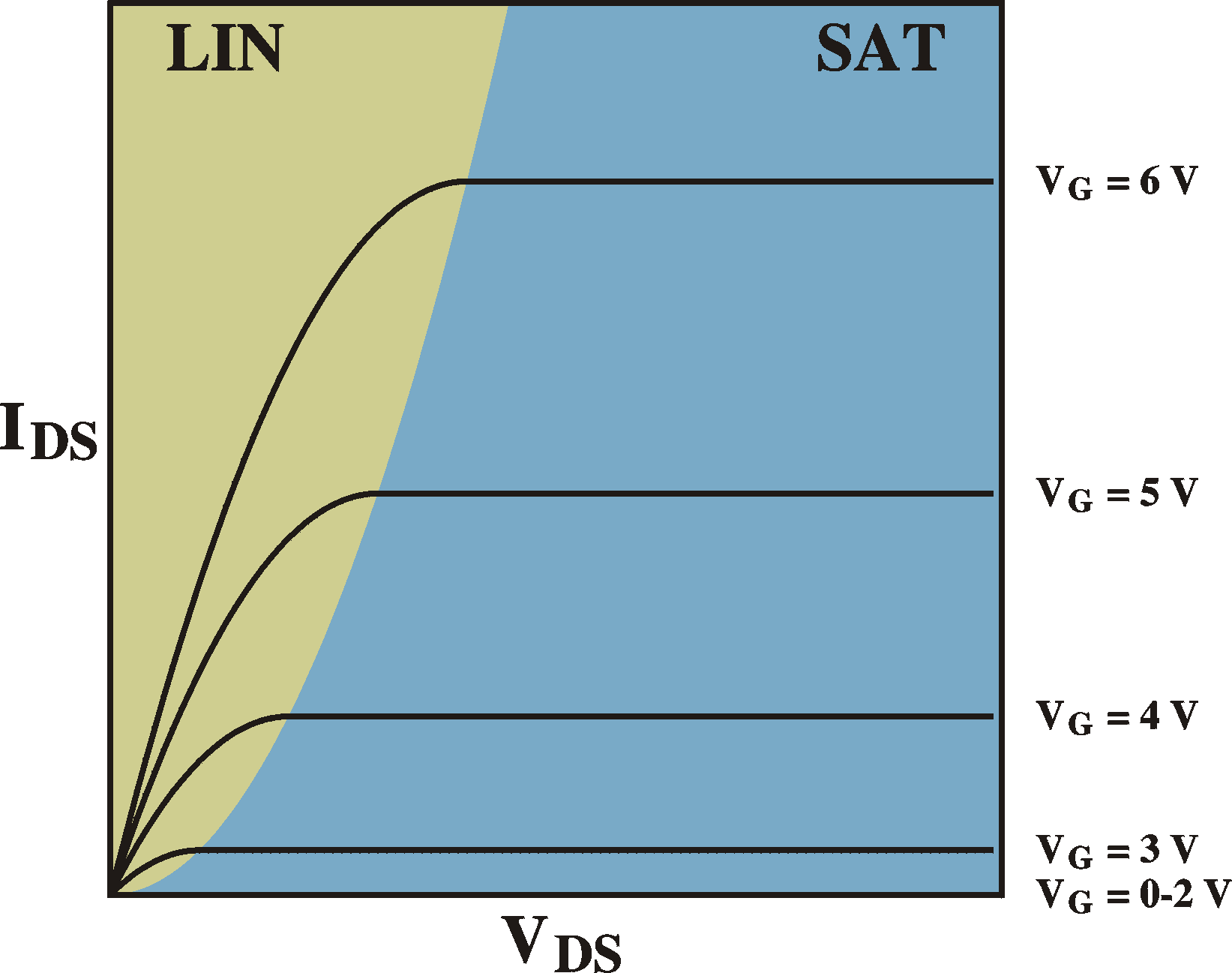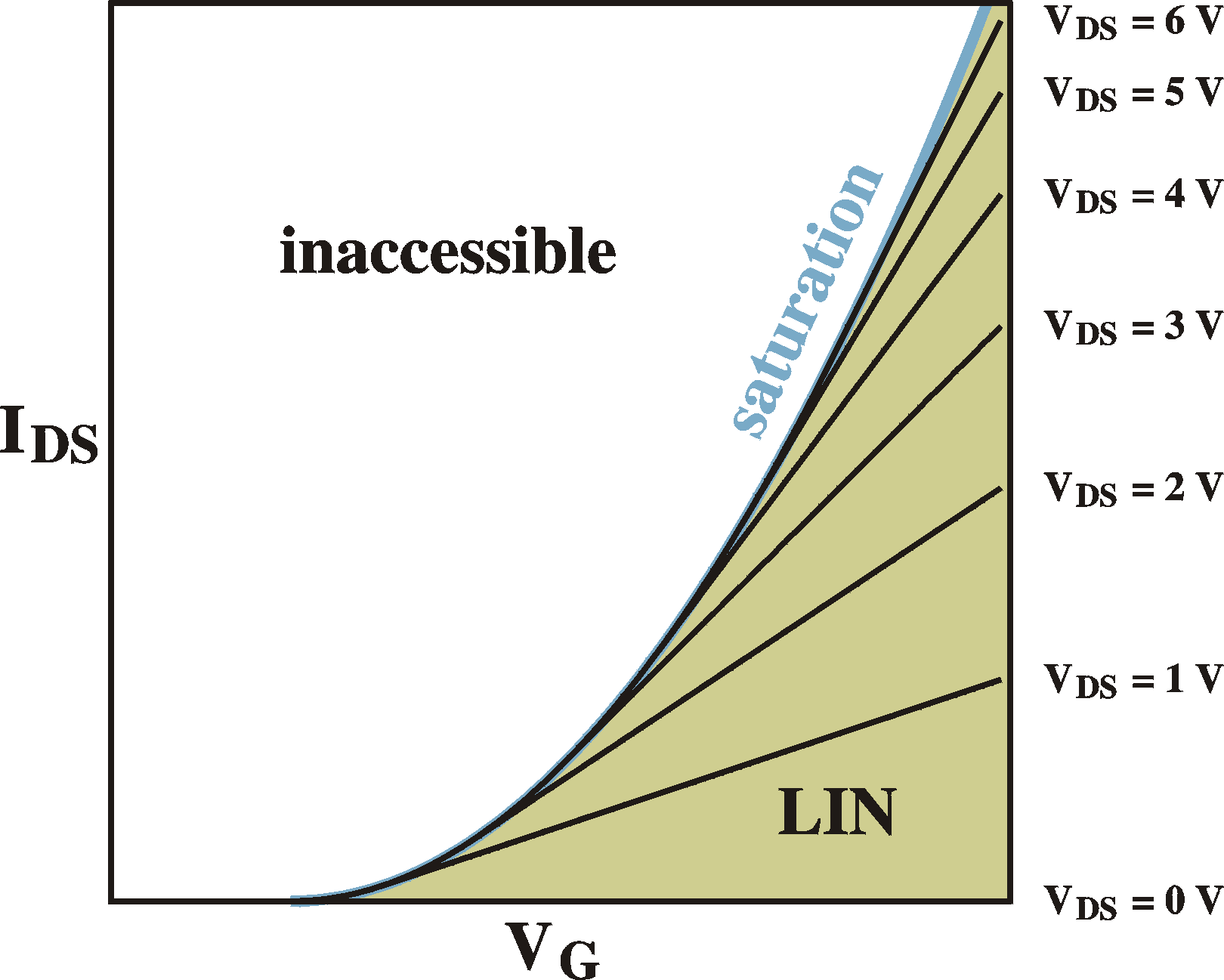Examples of IV curves (IDS vs. VDS; left) for different gate voltages and transfer curves (IDS vs. VG; right) for different drain-source voltages. VT = 2 V.

For completeness sake and for later reference, here are the charge distributions and voltage curves for the different regimes, ranging from 1) equilibrium, 2) linear regime, 3) onset of saturation, 4) in saturation.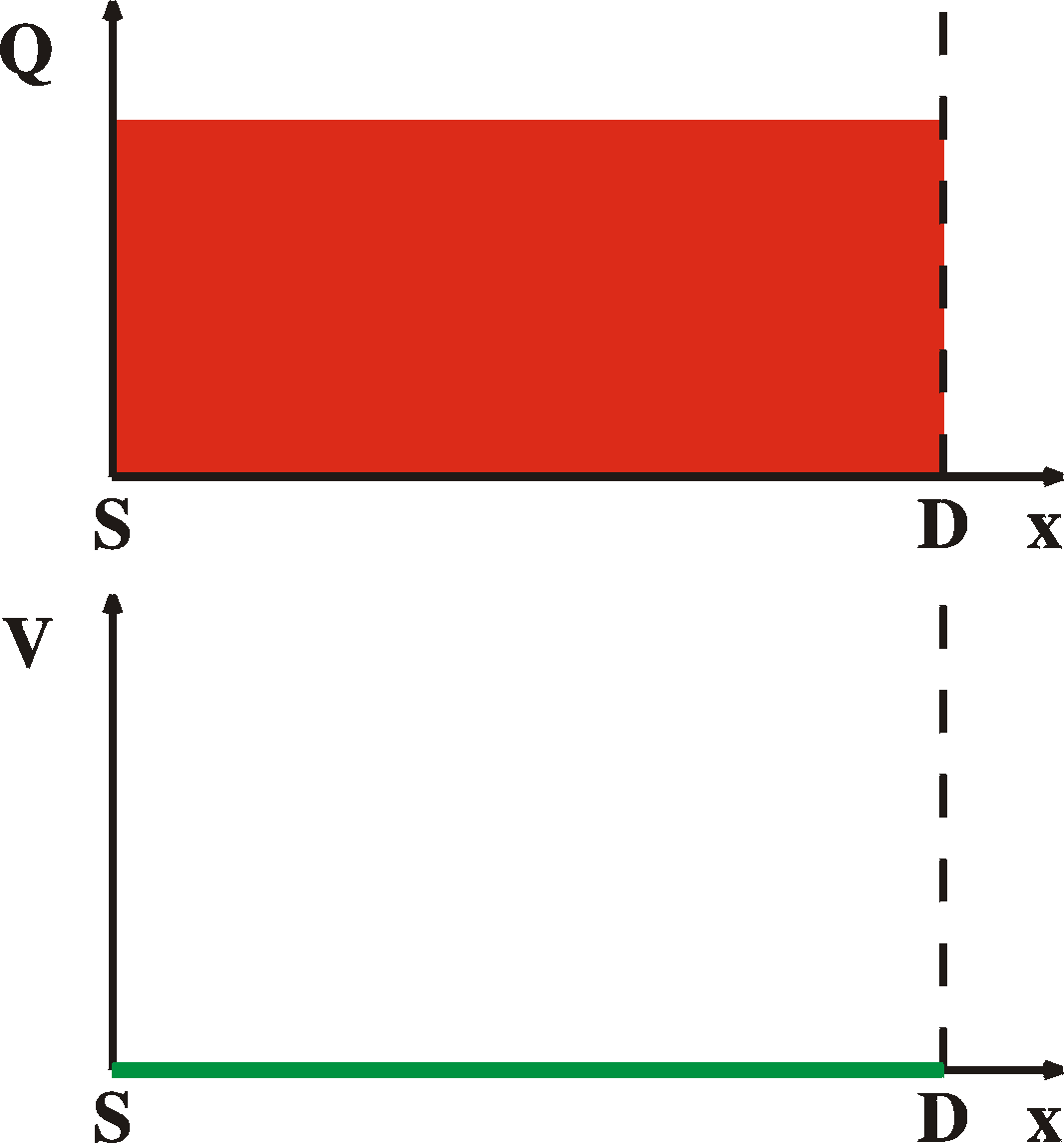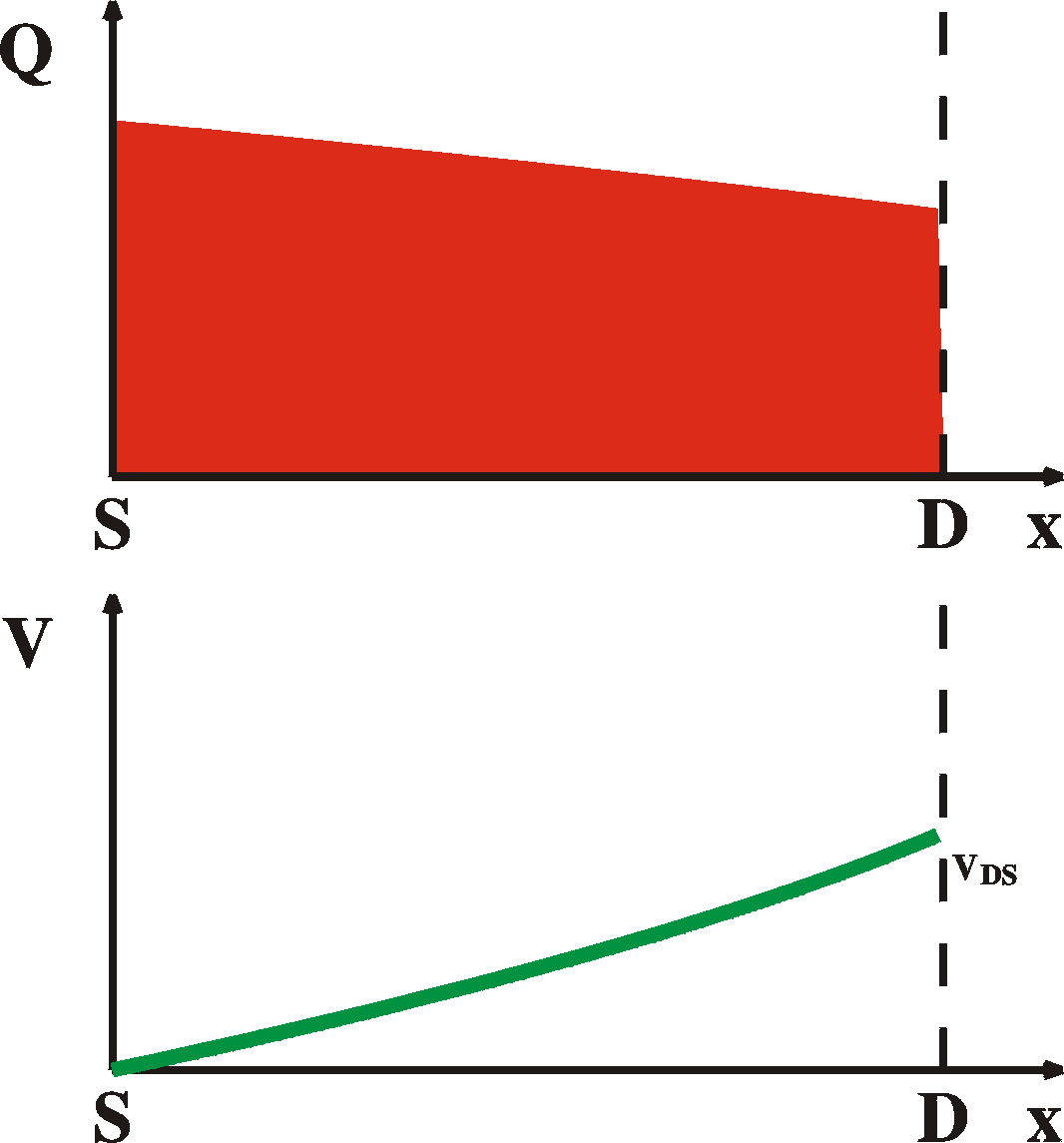VDS = 0 V VDS < VG-VT LIN VDS = VG-VT onset of SAT VDS > VG-VT SAT

## Contact resistance

In the above discussion it was assumed that the injection of carrier is not a limiting factor. In other words the contacts were considered low-ohmic However, the contacts can cause a severe barrier for the injection of carriers. The most obvious one is when at the electrodes a high-resistive region is formed. This causes the current to grow sub-linearly in the linear region. This can easily be shown in a simulation.
For strong currents, the contact resistance can become the limiting factor and the current saturates and becomes independent of VG: We have to imagine that the FET is made up of two contact resistances (2Rc) and the channel resistance, connected in series. Initially the current grows linear with VG (as explained by the text above). The channel resistance is thus proportional to 1/VG.For large VG the channel resistance disappears and the current settles at VDS / 2Rc.
More exact: when the current is increasing, the contact resistance induces a voltage drop at the source, Vs = Ids*Rc, the field at the source (Vgs) is reduced and the current drops (external observables in bold):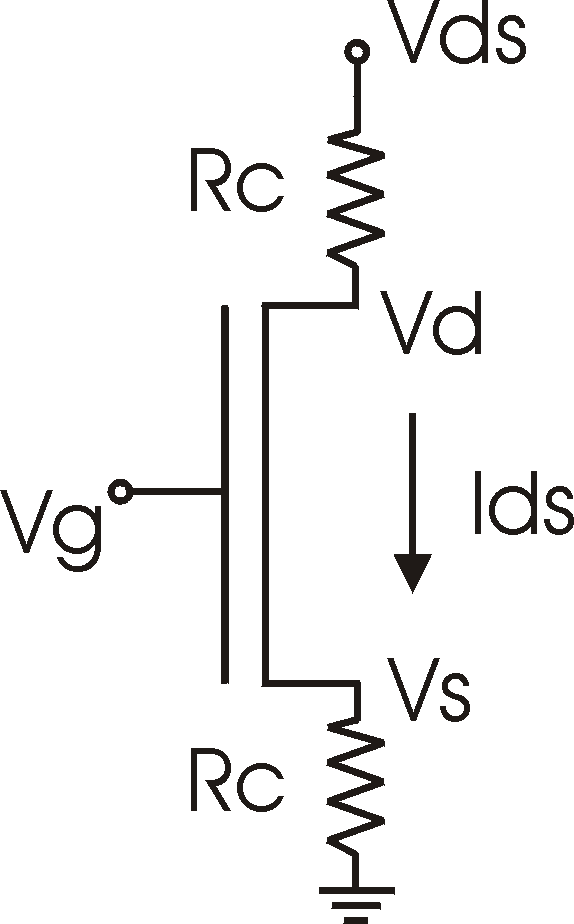Vs = IdsRc      Vd = Vds - Ids Rc      Vgs = Vg-Vs      Ids = mCoxZ/L (Vgs -VT) (Vd -Vs )

Simulation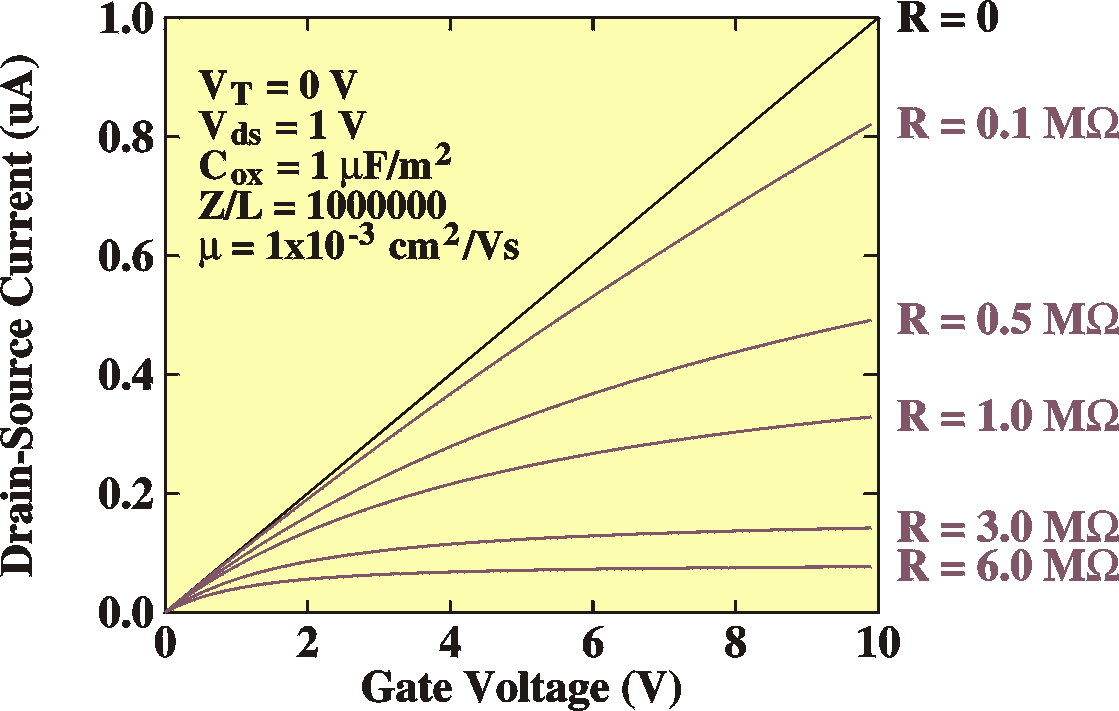Transfer curves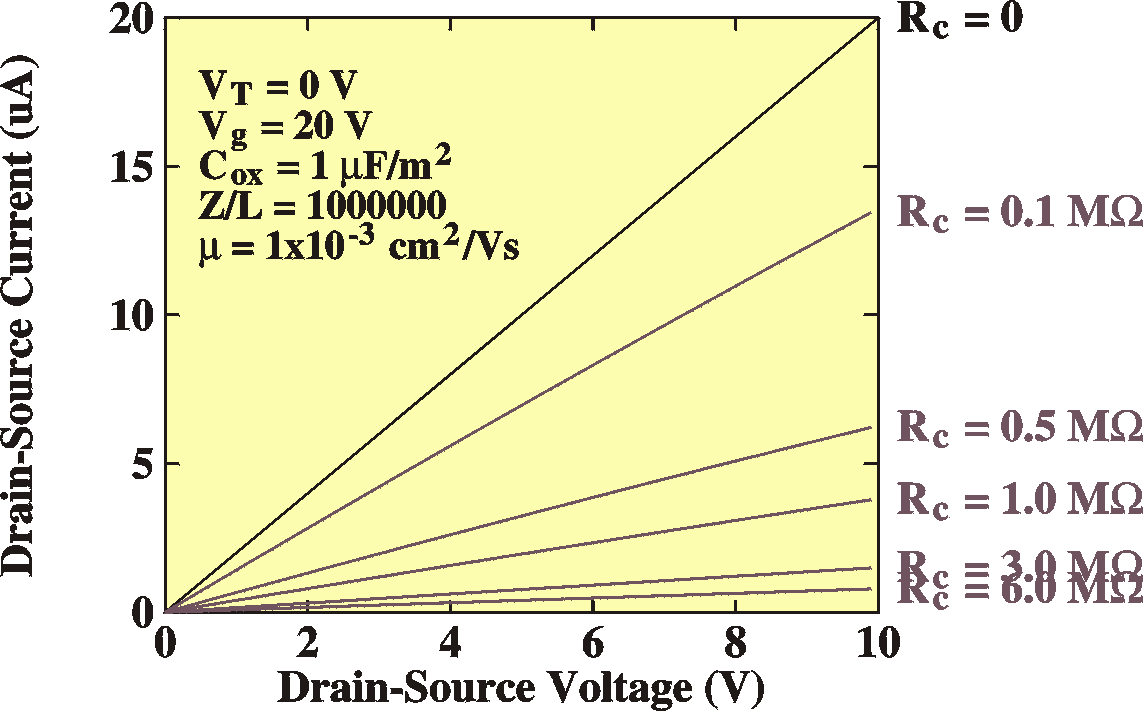IV Curves

## Other contact effects

The interface regions are not necessarily ohmic, as described above. Contacts are normally made of metal and the contact of this metal with a semiconductor might result in a Schottky diode. When a depletion layer is formed between the electrodes and the semiconductor, the current, might be limited by this Schottky barrier, see the bipolar device theory pages. However, these effects can be easily minimized by chosing a suitable electrode material. Normally, gold makes a good low-ohmic contact with p-type organic semiconductors.
In case the devices do have depletion regions at the electrodes, one side effect of this is that the saturation region doesn't saturate. This is especially pertinent for short channels, where the size of the depletion regions can be comparable to the channel length. Remember that the depletion width depends on the bias. In this case the bias is the drain-source voltage. When the bias is changed, the depletion width is changing. The channel (the rest of the distance between drain and source not filled by the depletion zone) is changing and the current can change, even in the saturation region.
In the extreme case, the depletion zones of the source and the drain can overlap. In this case, the entire area between the source and drain is depleted and we enter the space-charge-limited current regime.

## Stressing; threshold voltage shifts upon time

Stressing is the efffect that the threshold voltage VT changes with time when the bias is applied. It is not so difficult to imagine how this can occur:
As shown above, the current is linearly proportional to the density of free charges (Ids = a Qfree). These free charges are induced by the gate voltage and grow linear with VG once the threshold voltage has been overcome, Qfree = Cox(VG-VT). In other words, part of the gate voltage is not used to induce free carriers, but only serves to polarize the device and induce band bending.
After the free charges have been induced in the channel, they can be trapped onto deep localized states (Qfree becomes Qtrap). In these states they do no longer contribute to the current, while they still contribute to the induced charge: Qfree + Qtrap = Cox(VG-VT). The current therefore drops. Moving Qtrap to the other side of the equation, it is easy to show that effectively VT increases:
Ids = a Qfree = Cox[VG-(VT + Qtrap/Cox)]
Effectively, the threshold voltage has increased by Qtrap/Cox. In other words, we have to apply a larger bias to maintain the same current. The evolution of VT and Ids depends on the dsitribution of the trap states. In most cases the traps are not at discrete energy, but have a wide distribution. The currents drop therefore in a non-exponential way. Empirically, the best fit is obtained with (Stallinga 2004)
Ids= Ia exp[(t/t)a] + I0
The threshold-voltage shift is best described by a so-called stretched hyperbola (Gomes 2004)with Eth = kBT ln(nt).

## Organic FETs

Why doesn't this (always) work for our organic materials?

 The organic FETs are of the accumulation p-channel type. In the accumulation operation there is no space charge region caused by uncompensated ionized acceptors. All the space charge and band bending must come from intrinsic carriers - unbalanced holes-electrons which still follow pn = NCNVExp(-EG/kT), but p>n - and carriers in the strong accumulation channel. The result will be that we will still have the equations IDS    = Cox (VG -VT) mpVDS Z/L IDS = (1/2)m(Z/L) Cox (VG-VT)2 for currents through the strong accumulation channel in the linear and saturation regions, but that the threshold voltage doesn't follow the same equation anymore as given before. The threshold voltage now becomes (see Horowitz) VT = qNA/Cox However, see the chapter below about accumulation FETs. The FETs are thin-film transistors. Organic materials have a large sub-threshold voltage current. This also makes that the saturation currents become voltage dependent because the assumption that the size of the region where the voltage drops VG-VT is constant is no longer valid. In the discussion above it was assumed that when the device is in saturation, the rest of the voltage is absorbed in a very thin region. Such a strong field can be sustained becuase of the low carrier density. In organic FETs we have a situation where the free-carrier density below threshold is already substantial. The pinch-off region therefore has to be much larger and the rest of the voltage is then absorbed in a smaller region. This gives larger electric fields and larger currents. The currents still rise for increasing VDS. The mobilities are so low, that the above equations don't apply. For instance, we can have charge built-up at the electrodes which will limit the currents. In this case, the diffusion component of the current will take over in importance. See p. 443 of Sze. The assumption that the current is only depending on the electrical field is wrong. Instead of    I = qmenE we will have    I = qmenE + qDn dn/dx For low mobilities, the first term becomes small and the second dominating. Obviously, this becomes more important when we approach saturation because then we have the largest gradient in carrier concentrations, especially in the pinch-off region close to the drain (see the figures above). For low-mobility materials it is therefore advisable to measure in the linear region. Charge injection. In the above discussion it is assumed that the injection of carriers in the channel is not a limiting factor. In the classic n-type inversion-channel FET the source and drain electrodes are, in fact, made of n-type silicon. This means that, by the time an n-channel is created, the Fermi levels in the electrodes and in the channel have exactly lined up (by definition) and there is no barrier at the contact. Moreover, where there is no Fermi level alignement (outside the channel) there is a pn-junction that will prohibit conduction through anything else but the channel. We only have to worry about the charges inside the channel. In organic FETs the situation is different. When the p-channel is created, we still are not sure if we have Fermi level alignment between the gold electrodes and the p-channel. Maybe we have to overcome a Schottky barrier. This can be simulated by a diode in series with the FET. It implies that for low VDS the current is zero until VDS = Vbi.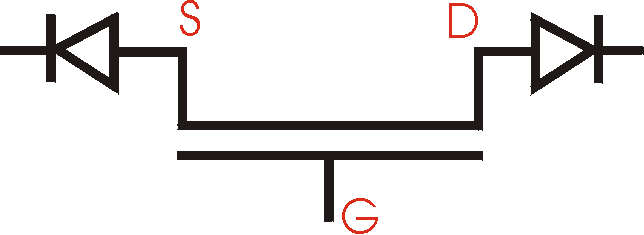The proposed structure is the one here on the left. This, however would never conduct (always one of the two diodes is closed). The best way to analyze this is with an MSM device (metal-semiconductor-metal, see p. 613 of Sze). The idea is that the depletion widths on opposite two Schottky barriers can start overlapping for certain voltages. When this happens, at VST, the currents rapidly rise and the barriers effectively disappear. The voltage at which the device opens is therefore NOT the built-in voltage of a diode, but rather the voltage at which breakdown occurs. See Fig. 40 on page 618 of Sze.  Of course, this is not really an MSM device, because we also have the gate. How does this relate to the FETs we are using?

## Accumulation-channel FET

For an accumulation channel FET, the free charge density also increases linearly with the gate, once the channel is formed. This is because we can apply the same reasoning as the one we used for the inversion channel FET: Once the channel is formed, we have a layer of free charges on both sides of the insulator, one on the metal side and one on the semiconductor side. This is equivalent to a capcitor, whose charge is equal to DVC, In this case Q = (VG-VT) Cox, namely, VT is lost from the externally applied voltage to induce the onset of the channel. Hoever, to calculate the threshold voltage is not easy. Horowitz uses a simplified idea by saying that the threshold voltage is that voltage that double the density of free carriers with respect to the carrriers caused by the doping. Without any bias, the charge density at any place of the device is qNA. The charge induced by the voltage is CoxVG, therefore, the threshold voltage can be defined as
VT = qNA/Cox
However, this is oversimplifying by assuming that the growth of free carriers before and after forming of the channel is equal, linear with the gate. In reality, the devices behave like normal FETs, with a turn-on voltage VT, after hich the current grows linear.

## Short-channel effects

For short channels we can expect the following:
Non saturating saturation currents. We assumed that the voltage drop along the channel is comprised of two parts, a gradual drop VG-VT independent of VDS over nearly the entire channel and the last part [VDS-(VG-VT)] completely absorbed by an infinitesimal small region. When the channel becomes shorter, the last part takes relatively more space and we cannot assume anymore that the voltage drop VG-VT occurs over a constant amount of space independent of VDS. In fact, this region becomes ever smaller and the field will proportionally increase, together with the currents. In other words, the current is, in saturation no longer independent of VDS. See Fig. 41 on p.478 of Sze.
We can easiliy explain this. At the source and the drain we have a depletion region (a zone without free holes in an n-p-n inversion channel FET). This zone will absorb the entire voltage drop VDS-(VG-VT). Normally these zones are thin compared to the bulk. It is easy to calculate the width of these depletion zones (see the section on Schottky barriers):
ys = [2es/qNA  (Vbi-ys)]1/2
yd = [2es/qNA  (Vbi-ys+VD)]1/2
for the depletion width at the source and drain respectively. Note that they also depend on the gate; once the channel has formed, the depletion width at the source disappears, but we still keep a depletion width at the drain which will absorp the excess VDS. Note that this "barrier" at the drain doesn't hinder the current, because it is in the forward direction for "minority carriers" (electrons for npn device).
When this depletion width becomes comparable to the channel length we can expect short-channel effects. This also depends on the acceptor concentration.
Non-zero subthreshold currents. Before threshold, we have pn-junctions on either side of the semiconductor. The depletion width W of these follows the standard calculations. When the channel length is decreased, these depletion regions can start overlapping. This will cause that the currents increase. This is easy to see why. When a carrier is injected by diffucsion over the first barrier,  into the "channel", it immediately starts feeling the fields of the second barrier on the other side and is pulled away to that electrode; it has a significant chance of making it to the other side. In fact, the diffusion (space-charge limited current) will dominate and the currents will be of the form J = VDS2.
Threshold voltage shift.
Because trapping of charges takes places, the current continuoasly drops. To compensate for this, a larger voltage has to be applied at the gate to keep the same amount of mobile charges in the channel.

## References

• Scientific American, July 1995.
• Physics of semiconductor devices, S.M. Sze, 2nd edition, John Wiley & sons, 1981, ISBN 0-471-05661-8
• Physics of semiconductor devices, M. Shur, Prentice Hall, 1990, ISBN 0136664962
• The Electrical Characterization of Semiconductors : Majority Carriers and Electron States (Techniques of Physics, Vol 14) by P. Blood, J.W. Orton, ASIN: 0125286279
• Advances in Sythetic Metals, Twenty years of Progress in Science and Technology. ed. P. Bernier, S. Lefrant and G. Bidan, Elsevier (1999).
• Fabrication and characterization of electronic devices based on poly(3-methylthiophene), H.L. Gomes, PhD thesis, University of Wales at Bangor, 1993.
• Electronic levels in MEH-PPV,  ICEL-2 Conference, Sheffield.  P. Stallinga, H.L. Gomes, H. Rost, A.B. Holmes, M.G. Harrison, and R.H. Friend. Accepted for publication in Synthetic Metals.
• Deep-level transient spectroscopy: A new method to characterize traps in semiconductors, D.V. Lang, J. Appl. Phys. 45, 3023 (1974).
• Charge carrier transport in poly(p-phenylenevinylene) light-emitting device, S. Forero, P.H. Nguyen, W. Bruetting, M. Schwoerer, Phys. Chem. Chem. Phys. 1, 1769 (1999).
• S. Karg, J. Steiger, and H. von Seggern, ICEL-2, proceedings, accepted for publication in Synthetic Metals (1999), and references therein.
• Microelectronic Circuits, A.S. Sedra, K.C. Smith, 4th edition, Oxford University Press, New York (1998).
• Electronic Devices and Circuits, T.F. Bogart Jr., 4th edition, Prentice Hall, Upper Saddle River (1997).
• Interface state mapping in terrylene, P. Stallinga, H.L. Gomes, M. Murgia, K. Müllen, Organic Electronics 3, 43 (2002).
• Electrical Characterization of FETs of sexithiophene, P. Stallinga, H.L. Gomes, F. Biscarini, M. Murgia, J.Appl.Phys. (2004).
• Gate voltage dependent mobility of oligothiophene field-effect transistors, G. Horowitz, R. Hajlaoui, D. Fichou, A. El Kassmi, J. Appl. Phys. 85, 3202 (1999).
• Theory of the field-effect mobility in amorphous organic transistors, M.C.J.M. Vissenberg, M. Matters, Phys. Rev. B 57, 12964 (1998).
• Organic field-effect transistors, G. Horowitz, Adv. Mater. 10, 365 (1998).
• Electrical properties of single crystals of rigid rodlike conjugated molecules, J.H. Schön, Ch. Kloc, R.A. Laudise, and B. Batlogg, Phys. Rev. B 58, 12952 (1998).
• Bias-induced threshold voltages shifts in thin-film organic transistors, H.L. Gomes, P. Stallinga, F. Dinelli, M. Murgia, F. Biscarini, D.M. de Leeuw, T. Muck, J. Geurts, L.W. Molenkamp, V. Wagner, Appl. Phys. Lett. 84, (2004).
• For the physical constants I relied on the very useful CERN pages
•        http://pdg.lbl.gov/2000/contents_sports.html,
It has the following official reference: C. Caso et al., The European Physical Journal C3 (1998) 1.
In the text the following variables and constants were used:
 variables variable description unit A area of the interface m2 b temperature scanning speed K/s C capacitance F C0 background capacitance or DC capacitance F DC capacitance-transient amplitude F cp hole capture rate 1/s e electrical permittivity F/m ep hole emission rate 1/s E electric field V/m Epa, EA, EA1, EA2, Eb activation energies J EF Fermi level J EVB, ECB valence-band level and conduction-band level J fm (metal) work function J G conductance (1/R) 1/W I current A J, J0 current density A/m2 NT, NA, ND impurity densities 1/m3 Q total space charge C R, Rb, Rd resistance W r charge density C/m3 S DLTS signal F
 variables variable description unit T temperature K t, t1, t2 time s t, t0 life time or decay time s Vbi built-in voltage V Vbb total band bending V V, VE, VF applied external voltage V Vn Fermi level depth in semiconductor relative to conduction band V W depletion width m w, w0 radial frequency rad/s x space coordinate m c electronaffinity V

 constants constant description value unit e0 permittivity of vacuum 8.854187817 ....10-12 F/m h Planck constant 6.6260755(40) 10-34 Js k Boltzmann constant 1.380658(12) 10-23 J/K q elementary charge 1.60217733(49) 10-19 C

Note: the units presented here are according to S.I.
In reality often different units are encountered. For
instance, energies are nearly always given in eV
(1.6 10-19 J) , depletion widths in nm or Å
and impurity levels in cm-3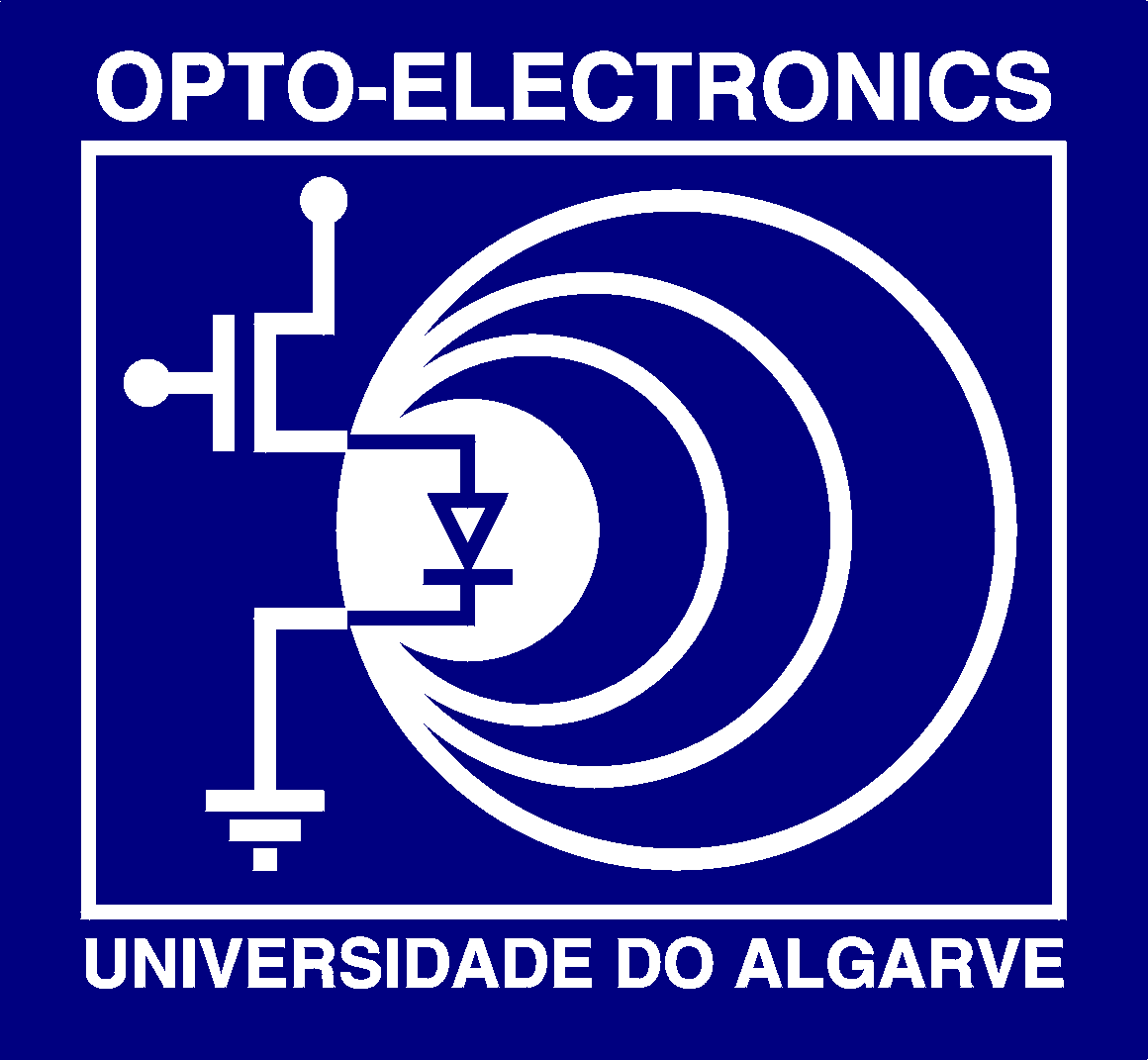page written and maintained by Peter Stallinga, pjotr@ualg.pt
Source written in HTML with NetScape 4.76. Pictures made with PjotrSoft (my software name), output in PostScript format. Converted with Corel Draw, GhostView, LViewPro and GIMP (Linux)  into GIFs.
In no way were any electrons harmed in the process!